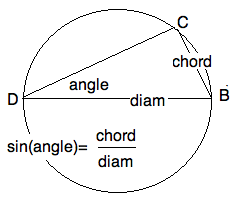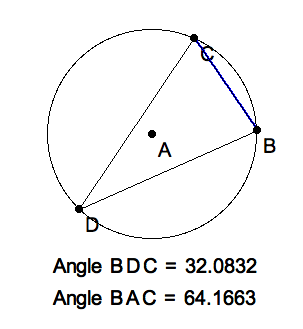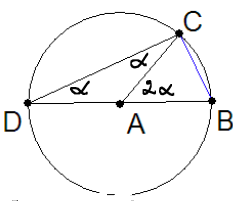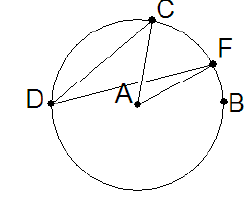Lesson: Rule of Sines, Cross Ratios, Perspective Rulers ======================================================= +++++++++++++++++++++++++++++++++++++++++++++++++ +++++++++++++++++++++++++++++++++++++++++++++++++ Introduction ------------ In this lesson we - Remind you of the Law of Sines - Show how the cross ratio is a perspective invariant - Apply this to create a ruler for perspective drawings The Law of Sines ---------------- From high school trigonometry you remember that the sine of an angle of a right triangle is defined as the ratio of the opposite side over the hypotenuse. From the calculus, you will recall that the sine is a differentiable function pass:[$\sin(\theta)$] from the reals to the interval pass:[$\[-1,1]$] equal to the x-coordinate of a point on the unit circle that has travelled a total arclength of pass:[$\theta$] units from pass:[$(1,0)$]. Recall that a line segment cutting across a circle is called a 'secant', and sometimes it is also called a 'chord' of the circle. [frame="none",cols="^",valign="middle", grid="none"] |=================== |pass:[] |The sine of an angle. |=================== .Definition. **************** The sine of an angle inscribed in a circle is the ratio of the chord to the diameter of the circle. The sign of the sine is that of the orientation of the angle: positive for counterclockwise. **************** The Greeks called the ratio of a secant pass:[$BC$] to the diameter pass:[$DB$] of a circle the 'chord' of the peripheral angle pass:[$\angle BDC$] 'subtending' the secant. As we shall see, this is also the sine of the angle. The word "chord" was translated into Arabic "jiba" ( from Sanscrit "jya" or "bow string") by the scholars in Baghdad who preserved Greek geometry during the Dark Ages. When Gherardo of Cremona (ca 1150) translated geometry from Arabic to Latin, he mistook the word for "jaib" or "bosom, fold in a garment, curve" in Latin, "sinus". [frame="none",cols="^",valign="middle",grid="all"] |=================== |pass:[] |Standard Labels |=================== This definition yields an easy proof of the .Law of Sines (LOS). ******************** Let pass:[$\triangle ABC$] have sides labeled pass:[$a, b, c$] and angles opposite the sides labeled pass:[$\alpha \beta \gamma$]. Then [frame="none",cols="^",valign="middle",grid="none"] |=================== | pass:[$\frac{\sin \alpha}{a}=\frac{\sin \beta}{b}=\frac{\sin \gamma}{c}$] |==================== **************** .Proof. ===================== From pass:[$\sin \alpha = \frac{a}{d}$] where pass:[$d$] is the diameter of the 'circumcircle' pass:[$\bigcirc ABC$], we see that the three ratios each equal the reciprocal of the diameter. Hence they are equal to each other. ===================== Peripheral Angle Theorem ------------------------ Of course, this proof begs the question: if the angle is transferred to a different circle, then the ratio of its new subtended secant to the new diameter is the same as the old. In particular, we would like to know that given a secant of a circle, every peripheral angle subtending this this secant has the same sine in the traditional sense. [frame="none",cols="^",valign="middle",grid="all"] |=================== |pass:[] |*Click image to download PAT.seg to explore it in KSEG.* |=================== .Peripheral Angle Theorem (PAT). ******************************** Given the secant pass:[$BC$] of a circle, with pass:[$D$] any other point moving around the circle, then pass:[$\sin( \angle BDC )$] remains constant. ******************************** .Comment. =============== We will prove this theorem in 3 steps. The first depends on an 'older' theorem on exterior angles of Euclidean triangles. By "older", we mean it was proved closer to the postulates in Euclid's Elements. By keeping track of the age of theorems we avoid circular reasoning. =============== .Exterior Angle Theorem (EXT). ****************************** Extend the side pass:[$AB$] of a pass:[$\triangle ABC$] past pass:[$B$] to pass:[$D$]. I.e. pass:[$(ABD)$] are collinear. Then the exterior angle is the sum of the two oppostite interior angles [frame="none",cols="^",valign="middle",grid="none"] |=================== | pass:[$\angle DBC = \angle BAC + \angle ACB$] |==================== **************** Proof of EXT ~~~~~~~~~~~~ You should use ruler and compass or KSEG to draw the figure being described here. We purposely do not include a picture of it so that you can practice following a description of a figure. Double the median pass:[$A A'$] to pass:[$E$] beyond the midpoint pass:[$A'$] of pass:[$BC$], and connect pass:[$E$] to pass:[$B$]. For reference, label a point on pass:[$(AB)$] beyond pass:[$B$], i.e. so that pass:[$B$] is between pass:[$A$] and pass:[$D$]. Because [frame="none",cols="^",valign="middle",grid="none"] |=================== |pass:[$A A' \cong EA'$] |pass:[$\angle A A'C \cong \angle EA'B$] |pass:[$C A' \cong BA'$] |==================== We have that pass:[$\triangle A A' C \cong \triangle EA'B$]. Therefore pass:[$\angle ACA' \cong \angle EBA'$] and pass:[$CA || BE$]. But then pass:[$\angle BAC \cong \angle DBE$] and we're done. .Question 1. ======================== What happens if the triangle is isosceles, with pass:[$|B-A|=|B-C|$]? ======================== +++++++++++++++++++++++++++
] Proof of PAT when angle grazes the center ~~~~~~~~~~~~~~~~~~~~~~~~~~~~~~~~~~~~~~~~~ We can imagine the problem to be that of finding a good place to sit in a loge of in a circular theater. 'La Scala' and Schakespeare's 'Globe Theater' had its loges arranged in a circular wall. By the PAT, every loge occupant saw the stage (a secant) with the same angle, hence the same apparent width. Of course, the distortion could not be avoided, and the queen still sat directly in the royal loge opposite the stage. [frame="none",cols="^",valign="middle",grid="all"] |=================== |pass:[] |=================== Let pass:[$A$] be the center of the circle, a lie on angle-leg pass:[$DB$]. By the EXT, the peripheral angle is half the central pass:[$\angle BAC$]. Since pass:[$\triangle DCB$] is inscribed in a semicircle, it is a right triangle, and pass:[$\sin D = \frac{CB}{diameter}$], justifying the two Greek definitions of the sine of an angle. It also proves .Corollary. ============== The length of a secant of a circle is the diameter times the sine of half the central angle it subtends. ============== Proof of PAT when angle sees the center ~~~~~~~~~~~~~~~~~~~~~~~~~~~~~~~~~~~~~~~ [frame="none",cols="^",valign="middle",grid="all"] |=================== |pass:[] |*Click image to download PAT.seg to explore it in KSEG.* |=================== This means, that pass:[$A$] lies inside the angle. Draw the diameter pass:[$DAE$] and observe that we have two cases of the first case, one above this diameter, and one below. Thus [frame="none",cols="^",valign="middle",grid="none"] |=================== |pass:[$\angle BDC = \angle BDE + \angle EDC = \frac{1}{2} (\angle BAE + \angle EAC) = \frac{1}{2} \angle BAE$] |==================== [frame="none",cols="^",valign="middle",grid="all"] |=================== |pass:[] |*Click image to download PATadder.seg which opens in KSEG.* | |=================== .Exercise 1. ===================== Apply a similar argument, with subtraction replacing addition of periferal and central angles for the last case, that the periferal angle does not see the center. ===================== [frame="none",cols="^",valign="middle",grid="all"] |=================== |pass:[] |=================== So, we have exhaustively proved PAT. You should note that we have used exclusively Euclidean arguments, very possibly the proof that Euclid wrote in his Elements. .Question 2. ======================== What happens when the periferal angle dips behind the stage? ======================== +++++++++++++++++++++++++++
+++++++++++++++++++++++++++ Suddenly the central angle becomes greater than a straight angle. That is, the periferal angle become obtuse. But since the two central angles add up to pass:[$2 \pi$], any two periferal angles, one "in front" and one "behind" the secant "stage", become supplementary. But recall, supplementary angles have the 'same' sine. pass:[
] The Cross Ratio is a Perspective Invariant ------------------------------------------ .Definition. ================ The 'cross ratio', pass:[$CR(A,B,C,D$], of four (distinct) collinear points, is pass:[$CR(A,B,C,D) = \frac{A-B}{A-D} \frac{C-D}{C-B}$] ================ .Definition. ================== The 'cross ratio', pass:[$CR(a,b,c,d)$], of four (distinct) concurrent lines, is pass:[$CR(a,b,c,d) = \frac{\sin \angle ab}{\sin \angle ad} \frac{\sin \angle cd}{\sin \angle cb}$] ================== Note the direction in which we measure a segment matters here, pass:[$\frac{A-B}{A-D} = - \frac{B-A}{A-D}$], and so does the orientation in which we measure an angle, pass:[$\sin \angle ab = - \sin \angle ba$]. However, for two crossing lines, it doesn't matter which of the two angles we use; being supplementary, their sins are the same. For notational symmetry, we have measured the angles clock-wise. It doesn't matter, as long as are consistent. Changing the sign of an angle changes the sign of its sin, but not the ratio of two angles whose sign was reversed. See how it would have been better if Gherardo had used the word "chord". On the other hand, "cochord" might sound worse than "cosine". [frame="none",cols="^",valign="middle",grid="all"] |=================== |pass:[] |=================== .Cross Ratio Theorem (CRT). *************************** Let pass:[$A, B, C, D$] be four distinct collinear points, and pass:[$P$] be any point not on that line. The their cross ratio depends only on the angles between the concurrent lines pass:[$a,b,c,d$] where pass:[$a = (PA), b= (PB), c= (PC), d=(PD)$]. That is pass:[$CR(A,B,C,D) = CR(a,b,c,d) (**)$]. *************************** Proof of CRT ~~~~~~~~~~~~ First, we abbreviate pass:[$sin AB = \sin \angle APB = \sin \angle ab$] etc. That would be the angles at pass:[$P$]. Second, we abbreviate pass:[$\sin B = \sin \angle PBA = \sin \angle CBP$] because these two angles are supplementary, and so have the same sines. Finally, we show that two numbers are equal by showing that their ratios equals pass:[$1$]. [frame="none",cols="^",valign="middle",grid="none"] |=================== |pass:[$\frac{CR(A,B,C,D)}{CR(a,b,c,d)}$] |pass:[$= \frac{ \frac{A-B}{A-D} \frac{C-D}{C-B} }{ \frac{sin AB}{sin AD}\frac{sin CD}{sin CB} }$] |pass:[$= \frac{ \frac{A-B}{sin AB} \frac{C-D}{sin CD} }{ \frac{A-D}{sin AD}\frac{C-B}{ sin CB} }$] |pass:[$= \frac{ \frac{P-A}{sin B} \frac{P-C}{sin D} }{ \frac{P-A}{sin D}\frac{P-C}{ sin B} }$] |pass:[$= \frac{ \frac{P-A}{P-A} \frac{P-C}{P-C} }{ \frac{sin B}{sin D}\frac{sin D}{sin B} }$] |pass:[$= 1$]. |==================== The rearrangement of the fractions has to be undertood carefully. Since everything is happening on the same line, we have replaced the vector pass:[$A-D$] by its signed length (the direction matters!). Now we apply the Law of Sines judiciously. Can you find the four triangles in which we replace ratios? This time we are allowed to replace vectors by their signed length because all four are concurrent. This is a little subtle, but has to be said here for completeness. .Comment. =================== Pretty neat, huh? Mathematicians die for clever arguments like this. The alternative is a considerable longer. While each step of the un-clever argument might be easier to see, there are so many of them that you loose the forest for the trees. =================== Two sets of four collinear points, pass:[$(ABCD), (A'B'C'D')$] are said to be 'in perspective position', if the four lines pass:[$(A'A) (B'B) (C'C) (D'D)$] are concurrent. s .Perspective Invariance Theorem (PIT). *************************************** Two cross ratios of four collinear points in perspective position are numerically equal. ************************* Proof of the PIT ~~~~~~~~~~~~~~~~ With pass:[$a=(A'A)$] etc, observe that [frame="none",cols="^",valign="middle",grid="none"] |=================== |pass:[$CR(A,B,C,D) = CR(a, b, c, d) = CR (A',B',C',D')$]. |==================== Perspective Rulers ------------------ By a 'ruler' we mean a line with a copy of the real numbers marked on it. Recall from high school, that pass:[$\ell = (AB)$] can be given a ruler by assignin pass:[$A |-> 0$] and pass:[$B |-> 1$]. In particular, we can find all multiples and fractions of unity by ruler and compass. Multiples are easy. .Question 3. ======================== How would you subdivide the segment pass:[$AB$] in 7 equal pieces? ======================== +++++++++++++++++++++++++++
+++++++++++++++++++++++++++ If instead of 7 we wanted some power of 2, then taking repeated halves would solve our problem. In fact, by just marking fractions with powers of two in their denominator (think of the inch-system) would work quite nicely in the real world. Such fractions are also called 'binary fractions'. We can approximate every real number by a sequence of convergent binary fractions. But to get an accurate, and for that matter quicker, answer we can use a similarity theorem from high school geometry. You'll need pencil and paper to follow along. On another line pass:[$AC$] extend pass:[$AC$] seven times to pass:[$D$]. Now pass:[$AC = \frac{1}{7} AD$]. Now draw pass:[$BD$] and a line parallel to pass:[$BD$] through every multiple of pass:[$AC$] on the helper line. These mark off seven equal pieces on the given segment pass:[$AB$]. pass:[
] .Comment. =============== The previous construction is actually a case of PIT, if we understand 'mutually parallel' as 'concurrent at infinity'. Although it is true, we have not proved PIT for this extended case. =============== Now, finally, consider a ray in the canvas at pass:[$V$] and two points, pass:[$Q I$] on the ray. Thus in the Euclidean plane of the canvas pass:[$(QIV)$]. This represents an infinite line in space, but in perspective. Equally spaced points on the space line would not be drawn with equally spaced points in perspective. The points have to get more and more close to each other as they approach the vanishing point pass:[$V$]. To find these milestones you may choose any convenient point pass:[$P$] in the canvas, but not on the line pass:[$(QI)$]. We shall call pass:[$P$] a 'lens'. Now choose any other line parallel pass:[$s$] to pass:[$(PV)$] that is convenient to work with. We shall call pass:[$s$] a scale for the perspective line pass:[$(VIQ)$]. Now transfer pass:[$Q, I$] to pass:[$s$] by drawing lines through pass:[$P$]. That is pass:[$Q' = (QP)s \and I' = (IP)s$]. .Question 4. ======================== Calculate pass:[$CR(X,Q,I,V) = CR(X',Q',I',V')$] ? ======================== +++++++++++++++++++++++++++
+++++++++++++++++++++++++++ Expanding, pass:[$\frac{X-Q}{X-V} \frac{I-V}{I-Q} = \frac{X'-Q'}{X'-V'} \frac{I'-V'}{I'-Q'}$] Notice the pass:[$V'$] does not exist. The line pass:[$(PV)$] is parallel to the scalin line pass:[$s$] and so meets it at pass:[$\infty$]. If we identify pass:[$X' = x, Q' = 0, I' = 1$] then we should also let pass:[$V'= \infty$]. Then the cross ratio becomse pass:[$\frac{x-0}{x-\infty}\frac{1-\infty}{1-0} = x$]. This applies a perspective ruler on the perspective line pass:[$(OQV)$]. Therefore, for two points pass:[$X(QV) \and Y(QV)$] we can assign the perspective distance pass:[$dist_{QV}(XY) = CR(X,Q,Y,V) = \frac{X'-Q'}{Y'-Q'}$]. pass:[
] [frame="none",cols="^",valign="middle",grid="all"] |=================== |pass:[] |===================